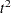ODS Graphics

Statistical procedures use ODS Graphics to create graphs as part of their output. ODS Graphics is described in detail in Chapter 21, Statistical Graphics Using ODS.

Before you create graphs, ODS Graphics must be enabled (for example, with the ODS GRAPHICS ON statement). For more information about enabling and disabling ODS Graphics, see the section Enabling and Disabling ODS Graphics in Chapter 21, Statistical Graphics Using ODS.

The overall appearance of graphs is controlled by ODS styles. Styles and other aspects of using ODS Graphics are discussed in the section A Primer on ODS Statistical Graphics in Chapter 21, Statistical Graphics Using ODS.

PROC CLUSTER can produce plots of the cubic clustering criterion, pseudo F, and pseudostatistics, and a dendrogram. To plot a statistic, you must ask for it to be computed via one or more of the CCC, PSEUDO, or PLOT options.

You can reference every graph produced through ODS Graphics with a name. The names of the graphs that PROC CLUSTER generates are listed in Table 30.5, along with the required statements and options.

Table 30.5 Graphs Produced by PROC CLUSTER

ODS Graph Name

Plot Description

Statement & Option

CubicClusCritPlot

Cubic clustering criterion for the number of clusters

PROC CLUSTER PLOTS=CCC

PseudoFPlot

Pseudo F criterion for the number of clusters

PROC CLUSTER PLOTS=PSF

PseudoTSqPlot

Pseudocriterion for the number of clusters

PROC CLUSTER PLOTS=PST2

CccAndPsTSqPlot

Cubic clustering criterion and pseudoPROC CLUSTER PLOTS=(CCC PST2)

CccAndPsfPlot

Cubic clustering criterion and pseudo F

PROC CLUSTER PLOTS=(CCC PSF)

CccPsfAndPsTSqPlot

Cubic clustering criterion, pseudo F, and pseudoPROC CLUSTER PLOTS=ALL

Dendrogram

Dendrogram
(tree diagram)

PROC CLUSTER
PLOTS=DENDROGRAM